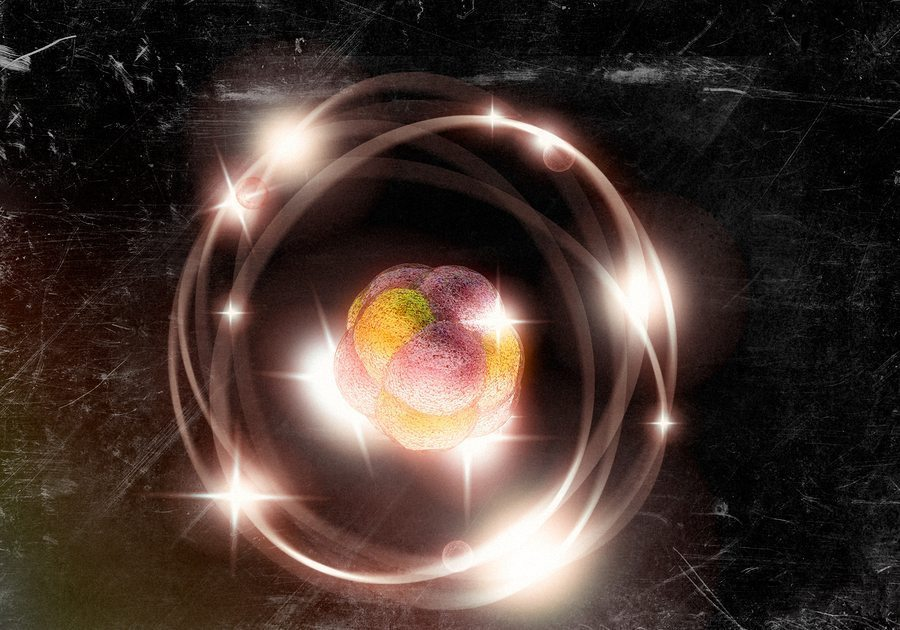Science Site

Quantum Chemistry

Published on Jun 1, 2016

Tags:Keywords:, , , ,When scientists conducted various kinds of experiments with atoms, they found that atoms actually behave unlike the bigger particles of matter. Scientists found that the explanations that were suggested by classical theory were inadequate and often wrong if the same principles were applied to the atoms. It was agreed that the behavior of the atom has to be studied differently and quantum chemistry was born. It is a relatively new branch of chemistry which is in particular concerned with the behavior of microscopic matter instead of macroscopic objects.

Schrödinger equation

Quantum chemistry was born when chemists tried to apply quantum mechanics to explain the chemical systems. The most crucial step is solving the equation that was put forth by the famous physicist Erwin Schrödinger. The solution of this equation helps in understanding how the electrons are distributed in the molecule. Unfortunately, the equation can only be accurately solved for the hydrogen atom since it involves only a single electron. For more complex systems, the chemists try to obtain only approximate solutions and then try to provide answers based on those solutions. When the electronic structure of a molecule is understood, it can be used to explain the chemical properties that are exhibited by the molecule. Quantum chemistry aims to define the principles which govern the nature of atoms and form a model which can help us provide a reason for all the observations concerning it.

Atomic behavior

Under quantum chemistry, a model was sought so that it was easier to predict as well as provide a rationale for the bizarre behavior of atoms. It was found that matter exhibits dual nature of a particle and a wave and acts as per the requirement. This model is referred to as the wave model because it is based on the wave-like properties of matter. The model states that the location of an electron inside an atom cannot be predicted accurately. There is always some uncertainty that is incorporated in the calculation. This is because the electrons exist not entirely as particles but in the form of “clouds” which have no fixed orbital rotation around the nucleus as it was thought before. According to quantum chemistry, everything has a value of probability associated with it and so it also dictates the probability of finding an electron in a particular part of space around the nucleus.

Quantum numbers

When the Schrodinger equation is solved, it provides a solution which depends on certain numbers called quantum numbers. These quantum numbers help define the space in which finding the probability of an electron will be high. Directly translated, these quantum numbers actually determine the orbital and sub-orbital that an electron would occupy. The principal quantum number is n, the secondary quantum number is l and the spin quantum number is s. n tells the orbital in which the electron is present, l provides information about the sub-orbital. It also helps in determining the angular momentum associated with the electron. The spin quantum number gives information about the orientation or spin of the electron.